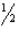/
/
/
36. A 20-g bullet moving at 1 000 m/s fired
Not my Question
Flag Content

# Question :   36. A 20-g bullet moving at 1 000 m/s fired : 2102139

36. A 20-g bullet moving at 1 000 m/s is fired through a one-kg block of wood emerging at a speed of 100 m/s. If the block had been originally at rest and is free to move, what is its resulting speed?

A. 9 m/s

B. 18 m/s

C. 90 m/s

D. 900 m/s

37. A 20-g bullet moving at 1 000 m/s is fired through a one-kg block of wood emerging at a speed of 100 m/s. What is the kinetic energy of the block that results from the collision if the block had not been moving prior to the collision and was free to move?

A. 10 kJ

B. 9.8 kJ

C. 0.16 kJ

D. 0.018 kJ

38. A 20-g bullet moving at 1 000 m/s is fired through a one-kg block of wood emerging at a speed of 100 m/s. What is the change in the kinetic energy of the bullet-block system as a result of the collision assuming the block is free to move?

A. 0 J

B. 9.7 kJ

C. -9.7 kJ

D. -18 J

39. An object of mass m moving at speed v0 strikes an object of mass 2m which had been at rest. The first object bounces backward along its initial path at speed v0. Is this collision elastic, and if not, what is the change in kinetic energy of the system?

A. The collision is elastic.

B. The kinetic energy decreases by mv2.

C. The kinetic energy decreases bymv2.

D. The kinetic energy increases by mv2.

40. A billiard ball is moving in the x-direction at 30.0 cm/s and strikes another billiard ball moving in the y-direction at 40.0 cm/s. As a result of the collision, the first ball moves at 50.0 cm/s, and the second ball stops. In what final direction does the first ball move?

A. in the x-direction

B. at an angle of 53.1? ccw from the x-direction

C. at an angle of 45.0? ccw from the x-direction

D. Such a collision cannot happen.

## Solution 5 (1 Ratings )

Solved
Physics 1 Year Ago 99 Views